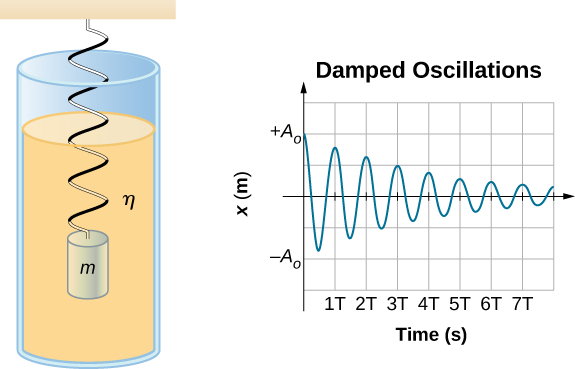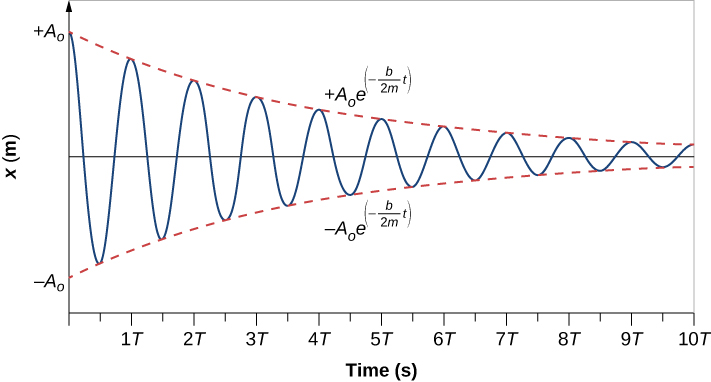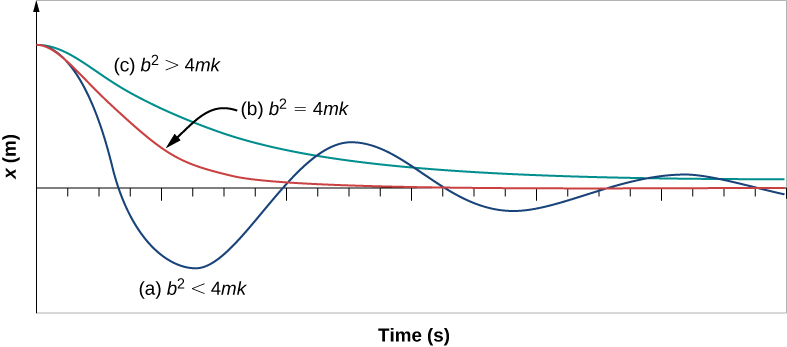Oscillations

# Damped Oscillations

### Learning Objectives

By the end of this section, you will be able to:

• Describe the motion of damped harmonic motion
• Write the equations of motion for damped harmonic oscillations
• Describe the motion of driven, or forced, damped harmonic motion
• Write the equations of motion for forced, damped harmonic motion

In the real world, oscillations seldom follow true SHM. Friction of some sort usually acts to dampen the motion so it dies away, or needs more force to continue. In this section, we examine some examples of damped harmonic motion and see how to modify the equations of motion to describe this more general case.

A guitar string stops oscillating a few seconds after being plucked. To keep swinging on a playground swing, you must keep pushing ((Figure)). Although we can often make friction and other nonconservative forces small or negligible, completely undamped motion is rare. In fact, we may even want to damp oscillations, such as with car shock absorbers.

To counteract dampening forces, you need to keep pumping a swing. (credit: Bob Mical)(Figure) shows a mass m attached to a spring with a force constant $k.$ The mass is raised to a position ${A}_{0}$, the initial amplitude, and then released. The mass oscillates around the equilibrium position in a fluid with viscosity but the amplitude decreases for each oscillation. For a system that has a small amount of damping, the period and frequency are constant and are nearly the same as for SHM, but the amplitude gradually decreases as shown. This occurs because the non-conservative damping force removes energy from the system, usually in the form of thermal energy.

For a mass on a spring oscillating in a viscous fluid, the period remains constant, but the amplitudes of the oscillations decrease due to the damping caused by the fluid.Consider the forces acting on the mass. Note that the only contribution of the weight is to change the equilibrium position, as discussed earlier in the chapter. Therefore, the net force is equal to the force of the spring and the damping force $\left({F}_{D}\right)$. If the magnitude of the velocity is small, meaning the mass oscillates slowly, the damping force is proportional to the velocity and acts against the direction of motion $\left({F}_{D}=\text{−}bv\right)$. The net force on the mass is therefore

$ma=\text{−}bv-kx.$

Writing this as a differential equation in x, we obtain

$m\frac{{d}^{2}x}{d{t}^{2}}+b\frac{dx}{dt}+kx=0.$

To determine the solution to this equation, consider the plot of position versus time shown in (Figure). The curve resembles a cosine curve oscillating in the envelope of an exponential function ${A}_{0}{e}^{\text{−}\alpha t}$ where $\alpha =\frac{b}{2m}$. The solution is

$x\left(t\right)={A}_{0}{e}^{-\frac{b}{2m}t}\text{cos}\left(\omega t+\varphi \right).$

It is left as an exercise to prove that this is, in fact, the solution. To prove that it is the right solution, take the first and second derivatives with respect to time and substitute them into (Figure). It is found that (Figure) is the solution if

$\omega =\sqrt{\frac{k}{m}-{\left(\frac{b}{2m}\right)}^{2}}.$

Recall that the angular frequency of a mass undergoing SHM is equal to the square root of the force constant divided by the mass. This is often referred to as the natural angular frequency, which is represented as

${\omega }_{0}=\sqrt{\frac{k}{m}}.$

The angular frequency for damped harmonic motion becomes

$\omega =\sqrt{{\omega }_{0}^{2}-{\left(\frac{b}{2m}\right)}^{2}}.$
Position versus time for the mass oscillating on a spring in a viscous fluid. Notice that the curve appears to be a cosine function inside an exponential envelope.Recall that when we began this description of damped harmonic motion, we stated that the damping must be small. Two questions come to mind. Why must the damping be small? And how small is small? If you gradually increase the amount of damping in a system, the period and frequency begin to be affected, because damping opposes and hence slows the back and forth motion. (The net force is smaller in both directions.) If there is very large damping, the system does not even oscillate—it slowly moves toward equilibrium. The angular frequency is equal to

$\omega =\sqrt{\frac{k}{m}-{\left(\frac{b}{2m}\right)}^{2}}.$

As b increases, $\frac{k}{m}-{\left(\frac{b}{2m}\right)}^{2}$ becomes smaller and eventually reaches zero when $b=\sqrt{4mk}$. If b becomes any larger, $\frac{k}{m}-{\left(\frac{b}{2m}\right)}^{2}$ becomes a negative number and $\sqrt{\frac{k}{m}-{\left(\frac{b}{2m}\right)}^{2}}$ is a complex number.

(Figure) shows the displacement of a harmonic oscillator for different amounts of damping. When the damping constant is small, $b<\sqrt{4mk}$, the system oscillates while the amplitude of the motion decays exponentially. This system is said to be underdamped, as in curve (a). Many systems are underdamped, and oscillate while the amplitude decreases exponentially, such as the mass oscillating on a spring. The damping may be quite small, but eventually the mass comes to rest. If the damping constant is $b=\sqrt{4mk}$, the system is said to be critically damped, as in curve (b). An example of a critically damped system is the shock absorbers in a car. It is advantageous to have the oscillations decay as fast as possible. Here, the system does not oscillate, but asymptotically approaches the equilibrium condition as quickly as possible. Curve (c) in (Figure) represents an overdamped system where $b>\sqrt{4mk}.$ An overdamped system will approach equilibrium over a longer period of time.

The position versus time for three systems consisting of a mass and a spring in a viscous fluid. (a) If the damping is small $\left(b<\sqrt{4mk}\right)$, the mass oscillates, slowly losing amplitude as the energy is dissipated by the non-conservative force(s). The limiting case is (b) where the damping is $\left(b=\sqrt{4mk}\right)$. (c) If the damping is very large $\left(b>\sqrt{4mk}\right)$, the mass does not oscillate when displaced, but attempts to return to the equilibrium position.Critical damping is often desired, because such a system returns to equilibrium rapidly and remains at equilibrium as well. In addition, a constant force applied to a critically damped system moves the system to a new equilibrium position in the shortest time possible without overshooting or oscillating about the new position.

Check Your Understanding Why are completely undamped harmonic oscillators so rare?

Friction often comes into play whenever an object is moving. Friction causes damping in a harmonic oscillator.

### Summary

• Damped harmonic oscillators have non-conservative forces that dissipate their energy.
• Critical damping returns the system to equilibrium as fast as possible without overshooting.
• An underdamped system will oscillate through the equilibrium position.
• An overdamped system moves more slowly toward equilibrium than one that is critically damped.

### Conceptual Questions

Give an example of a damped harmonic oscillator. (They are more common than undamped or simple harmonic oscillators.)

A car shock absorber.

How would a car bounce after a bump under each of these conditions?

(a) overdamping

(b) underdamping

(c) critical damping

Most harmonic oscillators are damped and, if undriven, eventually come to a stop. Why?

The second law of thermodynamics states that perpetual motion machines are impossible. Eventually the ordered motion of the system decreases and returns to equilibrium.

### Problems

The amplitude of a lightly damped oscillator decreases by $3.0%$ during each cycle. What percentage of the mechanical energy of the oscillator is lost in each cycle?

9%

### Glossary

critically damped
condition in which the damping of an oscillator causes it to return as quickly as possible to its equilibrium position without oscillating back and forth about this position
natural angular frequency
angular frequency of a system oscillating in SHM
overdamped
condition in which damping of an oscillator causes it to return to equilibrium without oscillating; oscillator moves more slowly toward equilibrium than in the critically damped system
underdamped
condition in which damping of an oscillator causes the amplitude of oscillations of a damped harmonic oscillator to decrease over time, eventually approaching zero